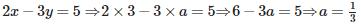Courses

# Important Questions (1 mark): Pair of Linear Equations in Two Variables

## 25 Questions MCQ Test Mathematics (Maths) Class 10 | Important Questions (1 mark): Pair of Linear Equations in Two Variables

Description
This mock test of Important Questions (1 mark): Pair of Linear Equations in Two Variables for Class 10 helps you for every Class 10 entrance exam. This contains 25 Multiple Choice Questions for Class 10 Important Questions (1 mark): Pair of Linear Equations in Two Variables (mcq) to study with solutions a complete question bank. The solved questions answers in this Important Questions (1 mark): Pair of Linear Equations in Two Variables quiz give you a good mix of easy questions and tough questions. Class 10 students definitely take this Important Questions (1 mark): Pair of Linear Equations in Two Variables exercise for a better result in the exam. You can find other Important Questions (1 mark): Pair of Linear Equations in Two Variables extra questions, long questions & short questions for Class 10 on EduRev as well by searching above.
QUESTION: 1

### A system of two linear equations in two variables has infinitely many solutions, if their graphs

Solution:

A system of two linear equations in two variables has infinitely many solutions, if their graphs coincide with each other

QUESTION: 2

### The area of the triangle formed by the lines 2x + y = 6, 2x – y + 2 = 0 and the x – axis is

Solution:

Here are the two solutions of each of the given equations. 2x+y = 6
2x+y=0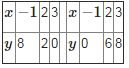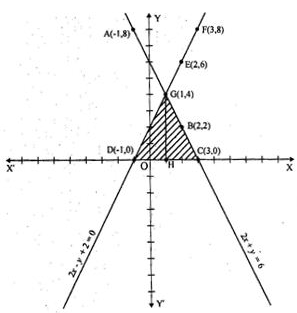The area bounded by the given lines and x−axis has been shaded in the graph.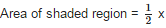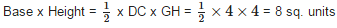QUESTION: 3

### The system of equations x – 4y = 8, 3x – 12y = 24

Solution:

Given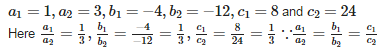Therefore, the system has infinite many solution.

QUESTION: 4

The system of linear equations a1x+b1y+c= 0 and a2x+b2y+c2 = 0 has a unique solution if

Solution:

The system of linear equations a1x+b1y+c= 0 and a2x+b2y+c2 = 0 has a unique solution if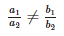QUESTION: 5

The sum of the numerator and denominator of a fraction is 18. If the denominator is increased by 2, the fraction reduces to 1/3. The fraction is

Solution: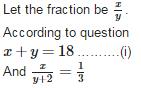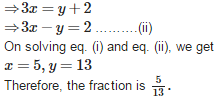QUESTION: 6

A system of two linear equations in two variables is consistent, if their graphs

Solution:

A system of two linear equations in two variables is consistent, if their graphs intersect only at a point, because it has a unitque solution.

QUESTION: 7

The vertices of the triangle formed by the lines x – y + 1 = 0, 3x + 2y – 12 = 0 and the x – axis are

Solution:

Here are the two solutions of each of the given equations.x−y+1=03x+2y−12=0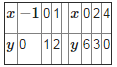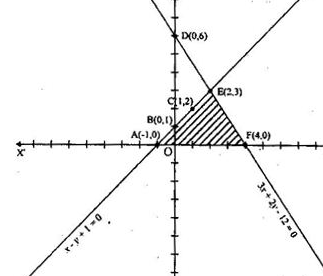The triangle formed by given two linear equations is shaded in the graph. The triangle formed by given lines is ΔAEF Therefore, the vertices of triangle AEF are A ( –1, 0), E (2, 3) and F (4, 0).

QUESTION: 8

The pair of equations 5x – 15y = 8 and 3x - 9y = 24/5 has

Solution:

Given: a1 = 5,a2 = 3,b1 = −15,b2 = −9,c1 = 8 and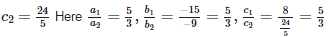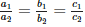Therefore, the the pair of given linear equations has infinitely many solutions.

QUESTION: 9

If x = α and y = β is the solution of the equations x – y = 2 and x + y = 4, then

Solution:

Given: x−y = 2…..(i)
And x+y = 4 ………(ii)
Adding eq. (i) and (ii) for elimination of y, we get
2x = 6 ⇒ x = 3
Putting the values of x in eq. (i), we get
3−y = 2 ⇒ y = 1
∴x = α = 3 and y = β = 1

QUESTION: 10

Half the perimeter of a rectangular garden, whose length is 4m more than its width is 36m. The area of the garden is

Solution:

Let the length of rectangular garden be x meters and breadth be y meters. According to question,
x = 4+y ⇒ x−y = 4 ……….(i)
Also half of the perimeter = 36 ⇒ x+y = 36 ……….(ii)
Adding eq. (i) and (ii), we get 2x = 40 ⇒ x = 20 meters
Subtracting eq. (i) from (ii), we get 2y = 32 ⇒ y = 16 meters
∴ Area = Length x Breadth = 20 x 16 = 320 sq. meters

QUESTION: 11

A system of two linear equations in two variables is dependentconsistent, if their graphs

Solution:

A system of two linear equations in two variables is dependentconsistent, if their graphs coincide with each other.

QUESTION: 12

One equation of a pair of dependent linear equations is – 5x + 7y = 2, then the second equation can be

Solution:

If equation of a pair of dependent linear equations, thenFor satisfying the condition of dependent linear equations, the values of a2,b2 and c2 should be the multiples of the values of a1,b1 and c1
∴ the values would be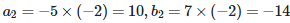and c1 = 2×(−2)=−4
∴ the second equation can be 10x−14y = −4

QUESTION: 13

The pair of equations x = 2 and y = – 3 has

Solution:

Here a unique solution of each variable of a pair of linear equations is given, therefore, it has one solution of a system of linear quations.

QUESTION: 14

The solution of px + qy = p – q and qx – py = p + q is

Solution: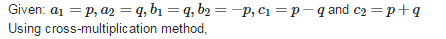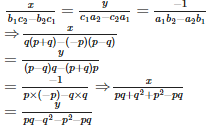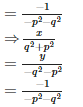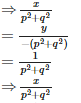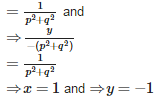QUESTION: 15

The difference between two numbers is 26 and one number is three times the other. The numbers are

Solution:

Let the two numbers be x and y.
According to question, x−y = 26 and x = 3y
Putting the value of x in x−y = 26 we get 3y−y = 26 ⇒ y = 13 And x = 3 × 13 = 39

QUESTION: 16

The lines representing the pair of equations 5x – 4y + 8 = 0 and 7x + 6y – 9 = 0

Solution: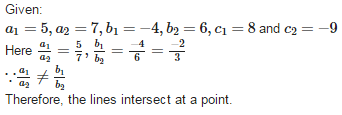QUESTION: 17

One equation of a pair of inconsistent linear equations is 2x – 3y = 4, then the second equation can be

Solution:

If equation of a pair of inconsistent linear equations, then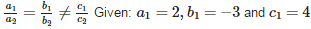For satisfying the condition of dependent linear equations, the values of a2,b2 and c2 should be the multiples of the values of a1,b1 and c1.
∴ the values would be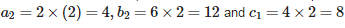∴ the second equation can be 4x−6y = 8

QUESTION: 18

The value of ‘k’ so that the system of equations 3x – 4y – 7 = 0 and 6x – ky – 5 = 0 have a unique solution is

Solution: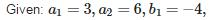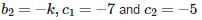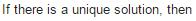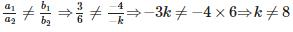QUESTION: 19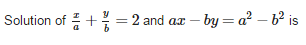Solution: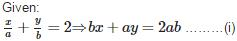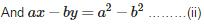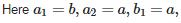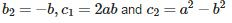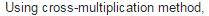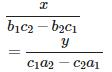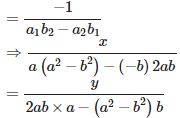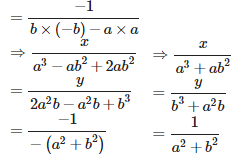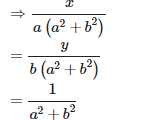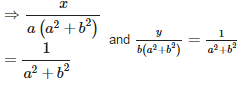QUESTION: 20

The sum of two numbers is 35 and their difference is 13. The numbers are

Solution:

Let the numbers be x and .y.
According to question, x+y = 35 ………(i) and x−y = 13 ………..(ii)
Adding eq. (i) and (ii), we get  2x = 48 ⇒ x = 24
Putting the value of x in eq. (i), we get 12+y = 35 ⇒ y = 13
Therefore, the numbers are 24 and 13.

QUESTION: 21

What will be the solution of these equations ax+by = a-b, bx-ay = a+b

Solution:
QUESTION: 22

The area of the triangle formed by x + 3y = 6, 2x – 3y = 12 and the y – axis is

Solution:

Here are the two solutions of each of the given equations. x+3y=62x−3y=12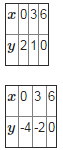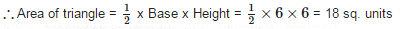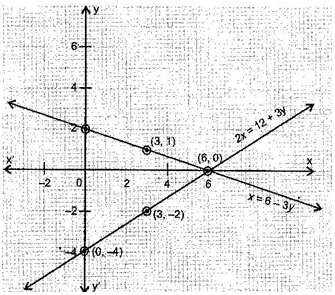QUESTION: 23

The value of ‘k’ so that the system of linear equations kx – y – 2 = 0 and 6x – 2y – 3 = 0 have no solution is

Solution: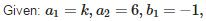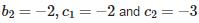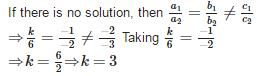QUESTION: 24

The pair of linear equations ax + by = c and px + qy = r has a unique solution then

Solution:

Given: a1 = a,a2 = p,b1 = b,b2 = q,c1 = c and c2 = r
Since the pair of given linear equations has a unique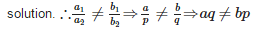QUESTION: 25

The value of ‘a’ so that the point (3, a) lies on the line represented by 2x – 3y = 5 is

Solution: